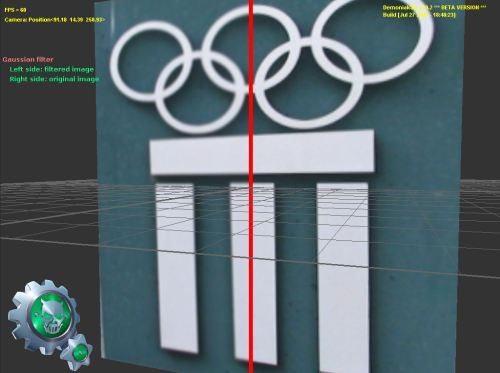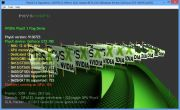Home Utilities Tutorials 3D Demos Graphics Cards Forums About

 GeeXLab
Current version: 0.45.1
>GeeXLab homepage

 FurMark
Current version: 1.30.0
>FurMark homepage

 GPU Caps Viewer
Current version: 1.55.0.0
>GPU Caps Viewer homepage

 GPU Shark
Current version: 0.26.0.0
>GPU Shark homepage

 Blogs
>JeGX's HackLab

 Geeks3D's Articles
>GPU Memory Speed Demystified

>How to Get your Multi-core CPU Busy at 100%

>How To Make a VGA Dummy Plug

>Night Vision Post Processing Filter

 PhysX FluidMark
Current version: 1.5.4
>FluidMark homepage

 TessMark
Current version: 0.3.0
>TessMark homepage

Current version: 0.3.0

 Demoniak3D
Current Version: 1.23.0
>Demoniak3D
>Libraries and Plugins
>Demos
>Codes SamplesImage Filtering with GLSL - Convolution Kernels By J�r�me Guinot - jegx [at] ozone3d [dot] net Initial draft: December 8, 2005 Last update: January 8, 2006 Translated from french by Samir Fitouri [ Index ]�Next Page2 - Gaussian FilterThis filter is used to add blur to an image.```1 2 1 2 4 2 1 2 1 ```The demo associated with this filter is DEMO_gaussian_filter.xml.[Vertex_Shader] void main(void) { gl_TexCoord = gl_MultiTexCoord0; gl_Position = ftransform(); } [Pixel_Shader] #define KERNEL_SIZE 9 // Gaussian kernel // 1 2 1 // 2 4 2 // 1 2 1 const float kernel[KERNEL_SIZE] = { 1.0/16.0, 2.0/16.0, 1.0/16.0, 2.0/16.0, 4.0/16.0, 2.0/16.0, 1.0/16.0, 2.0/16.0, 1.0/16.0 }; uniform sampler2D colorMap; uniform float width; uniform float height; const float step_w = 1.0/width; const float step_h = 1.0/height; const vec2 offset[KERNEL_SIZE] = { vec2(-step_w, -step_h), vec2(0.0, -step_h), vec2(step_w, -step_h), vec2(-step_w, 0.0), vec2(0.0, 0.0), vec2(step_w, 0.0), vec2(-step_w, step_h), vec2(0.0, step_h), vec2(step_w, step_h) }; void main(void) { int i = 0; vec4 sum = vec4(0.0); if(gl_TexCoord.s<0.495) { for( i=0; i0.505 ) { sum = texture2D(colorMap, gl_TexCoord.xy); } else { sum = vec4(1.0, 0.0, 0.0, 1.0); } gl_FragColor = sum; }Load the DEMO_gaussian_kernel.xml in Demoniak3D file and you will get the following result:Fig.2 - Gaussian filter.The working of the shaders in this tutorial is the following: the base image is plated on a mesh plane. The left part of the image (texture coordinate u < 0.495) is filtered while the right part (u > 0.505) is not. All the texels that are located in the [0.495; 0.505] interval will be colored in red in order to form a vertical separating line. The dimension of the base texture must be of a power of 2. In our case, they are 256x256. These measurements are passed as uniform variables to the pixel shaders in order to calculate the distances to access the neighboring pixels to the pixel being processed. The vertex and pixel shaders being the same for all the filters, only the convolution core will be given. If the code of the previous pixel shader does not work properly, it is possible to take of the branches and to unroll the loop as shown in the following code:[Vertex_Shader] void main(void) { gl_TexCoord = gl_MultiTexCoord0; gl_Position = ftransform(); } [Pixel_Shader] #define KERNEL_SIZE 9 // Gaussian kernel // 1 2 1 // 2 4 2 // 1 2 1 const float kernel[KERNEL_SIZE] = { 1.0/16.0, 2.0/16.0, 1.0/16.0, 2.0/16.0, 4.0/16.0, 2.0/16.0, 1.0/16.0, 2.0/16.0, 1.0/16.0 }; uniform sampler2D colorMap; uniform float width; uniform float height; const float step_w = 1.0/width; const float step_h = 1.0/height; const vec2 offset[KERNEL_SIZE] = { vec2(-step_w, -step_h), vec2(0.0, -step_h), vec2(step_w, -step_h), vec2(-step_w, 0.0), vec2(0.0, 0.0), vec2(step_w, 0.0), vec2(-step_w, step_h), vec2(0.0, step_h), vec2(step_w, step_h) }; void main(void) { int i = 0; vec4 sum = vec4(0.0); vec4 tmp = texture2D(colorMap, gl_TexCoord.st + offset); sum += tmp * kernel; tmp = texture2D(colorMap, gl_TexCoord.st + offset); sum += tmp * kernel; tmp = texture2D(colorMap, gl_TexCoord.st + offset); sum += tmp * kernel; tmp = texture2D(colorMap, gl_TexCoord.st + offset); sum += tmp * kernel; tmp = texture2D(colorMap, gl_TexCoord.st + offset); sum += tmp * kernel; tmp = texture2D(colorMap, gl_TexCoord.st + offset); sum += tmp * kernel; tmp = texture2D(colorMap, gl_TexCoord.st + offset); sum += tmp * kernel; tmp = texture2D(colorMap, gl_TexCoord.st + offset); sum += tmp * kernel; tmp = texture2D(colorMap, gl_TexCoord.st + offset); sum += tmp * kernel; gl_FragColor = sum; }All the previous codes run properly on a nVidia GPU based graphic controller. Graphics controllers featuring the ATI chipsets seem not to support the constant array functionality. In case of an ATI chipset, the pixel shader code becomes:[Pixel_Shader] #define KERNEL_SIZE 9 // Gaussian kernel // 1 2 1 // 2 4 2 // 1 2 1 float kernel[KERNEL_SIZE]; uniform sampler2D colorMap; uniform float width; uniform float height; float step_w = 1.0/width; float step_h = 1.0/height; vec2 offset[KERNEL_SIZE]; void main(void) { int i = 0; vec4 sum = vec4(0.0); offset = vec2(-step_w, -step_h); offset = vec2(0.0, -step_h); offset = vec2(step_w, -step_h); offset = vec2(-step_w, 0.0); offset = vec2(0.0, 0.0); offset = vec2(step_w, 0.0); offset = vec2(-step_w, step_h); offset = vec2(0.0, step_h); offset = vec2(step_w, step_h); kernel = 1.0/16.0; kernel = 2.0/16.0; kernel = 1.0/16.0; kernel = 2.0/16.0; kernel = 4.0/16.0; kernel = 2.0/16.0; kernel = 1.0/16.0; kernel = 2.0/16.0; kernel = 1.0/16.0; if(gl_TexCoord.s<0.495) { for( i=0; i0.505 ) { sum = texture2D(colorMap, gl_TexCoord.xy); } else { sum = vec4(1.0, 0.0, 0.0, 1.0); } gl_FragColor = sum; }The code of the pixel shader above is fully operational on a Radeon 9800pro graphics controller featuring the Catalyst 5.11 drivers. The accompanying projects are therefore provided in two versions: one exclusively intended for the nVidia chipsets while an other is meant for both ATI and nVidia.[ Index ]�Next Page

 GeeXLab demosGLSL - Mesh exploderPhysX 3 cloth demoNormal visualizer with GS